## 卷积神经网络理解 转

o
osc_g8254g7s

卷积神经网络（Convolutional Neural Network，CNN或ConvNet）是一种具有局部连接、权重共享等特性的深层前馈神经网络。我们通俗的印象在于卷积神经网络有利于图像处理。

如果用全连接前馈网络来处理图像时，会存在以下两个问题：

1）参数太多：如果你是一个100*100（RGB）图片，那么你的输入数目（输入神经元）就会有（30000），问题在于许多图片远远比这个大，如果是1000*1000（RGB）图片，那么输入层就会需要（3000000）个连接（神经元）,这无疑是一个很庞大的数字。每个连接都对应一个重参数。随着隐藏层神经元数量的增多，参数的规模也会急剧增加。这会导致整个神经网络的训练效率会非常低，也很容易出现过拟合。

2）局部不变形特征：自然图像中的物体都具有局部不变性特征，比如尺度缩放、平移、旋转等操作不影响其语义信息。而全连接前馈网络很难提取这些局

这种全连接的方法用在图像识别上面就显得太"笨"了，因为图像识别首先得找到图片中各个部分的"边缘"和"轮廓"，而"边缘"和"轮廓"只与相邻近的像素们有关。 卷积神经网络(CNN)就派上用场了，卷积神经网络可以简单地理解为，用滤波器(Filter)将相邻像素之间的"轮廓"过滤出来。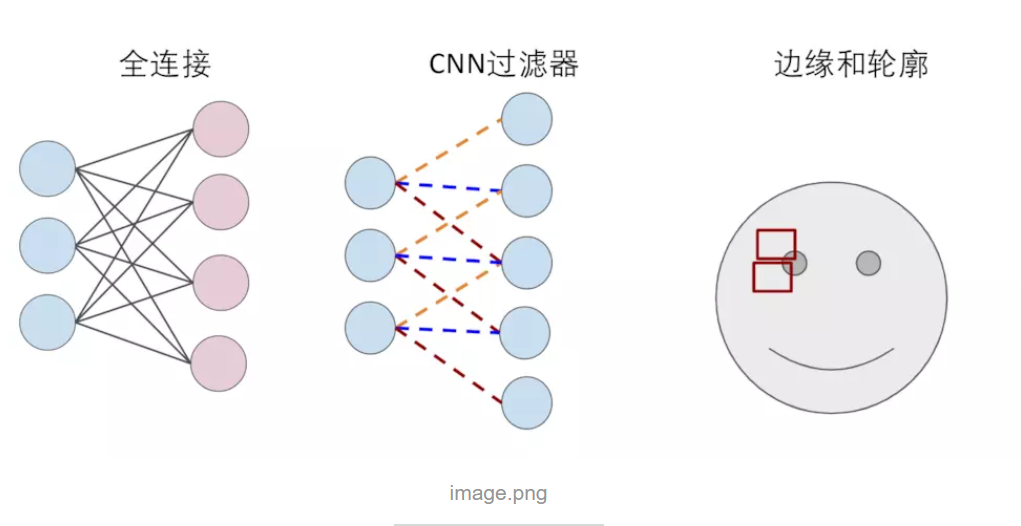目前的卷积神经网络一般是由卷积层汇聚层全连接层交叉堆叠而成的前馈神经网络，使用反向传播算法进行训练。接下来我们介绍卷积层。

1卷积层（convolution):

在原始的输入上进行特征的提取。特征提取简言之就是，在原始输入上一个小区域一个小区域进行特征的提取。

1.1:滤波器(卷积核）：

卷积的滤波器（Filter）是如何工作的呢？以下图，一个6x6的图片被一个3x3的滤波器（可以看成一个窗口）卷积为例，3x3的滤波器先和6x6的图片最左上角  的3x3矩阵卷积得到结果后，再向右移一步继续卷积（窗口滑动（stride）），直到将整个图片过滤完成，输出一个4x4的矩阵(图片)。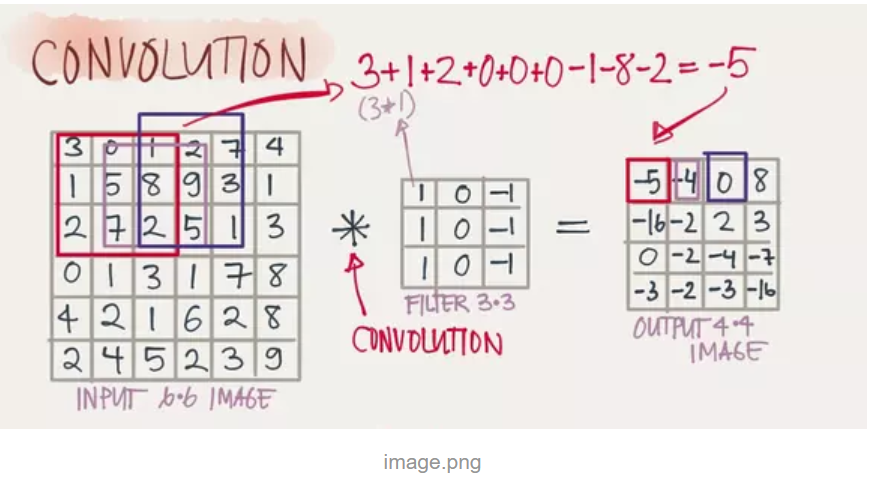这样做有什么意义？滤波器可以更好的把图像的“轮廓”给过滤出来，比如下图：依然是6*6的原图像（矩阵），图片有明显的竖直轮廓（10和0之间有一轮廓，这条轮廓需要被标记出来），中间为3*3的滤波器，映射出来的图像（右下第三个），比较好的展示了和原图像一样的特征（轮廓）。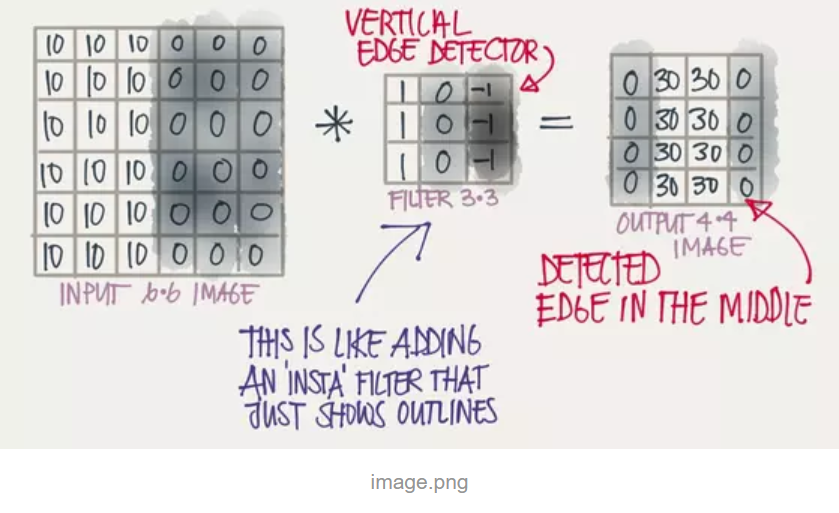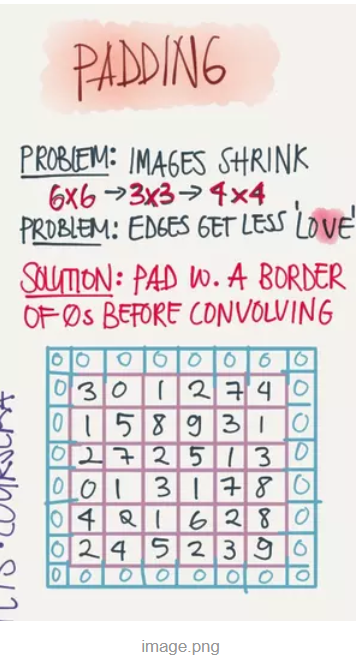1.3步长（Stride）：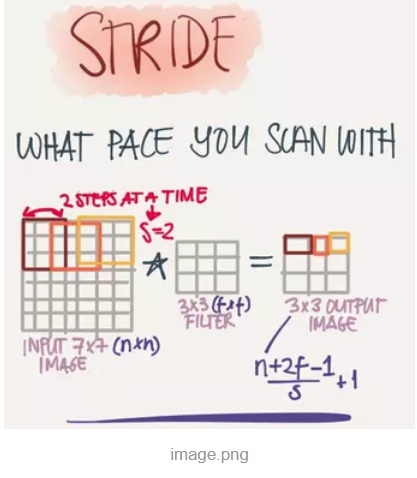### 1.4深度：

这里的深度是指输出层图片的深度，通常图片有红绿蓝(RGB)三个颜色通道(Channel)，那一个滤波器也需要三层滤波器对每个颜色通道进行过滤，于是6x6x3的图片经过3x3x3的滤波器过滤之后最终会得到一个4x4x1的图片，此时输出层图片的深度就是1。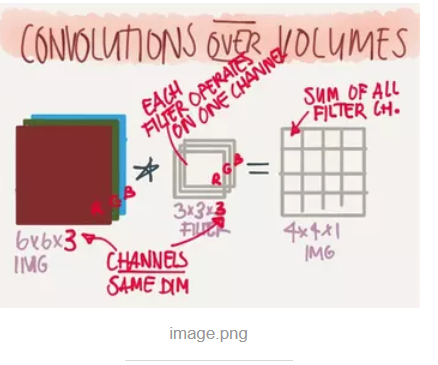增加滤波器的个数就能增加输出层图片的深度，同时滤波器的个数也决定了输出层图片的深度（两者相等）。下图两个3x3x3的滤波器将6x6x3的图片过滤得到一个4x4x2的图片。

2：卷积层计算图解：

知道原图像（矩阵）经过滤波器得到映射矩阵的计算过程吗，对于了解卷积神经网络是有好处的。我们以下图为例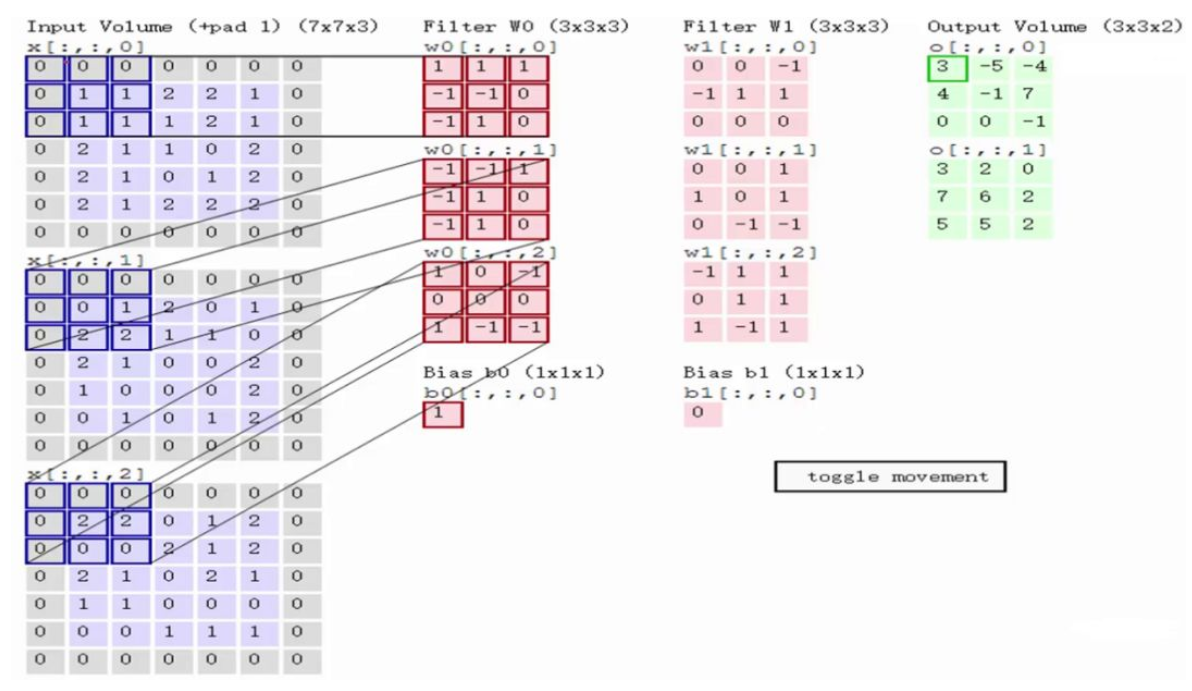注释：左区域的三个大矩阵是原式图像的输入，RGB三个通道用三个矩阵表示，大小为7*7*3。Filter W0表示1个filter助手，尺寸为3*3，深度为3（三个矩阵）；Filter W1也表示1个filter助手。因为卷积中我们用了2个filter，因此该卷积层结果的输出深度为2（绿色矩阵有2个）。Bias b0是Filter W0的偏置项，Bias b1是Filter W1的偏置项。OutPut是卷积后的输出，尺寸为3*3，深度为2。滤波器的值就是偏置项。

计算过程：

输入是固定的，filter是指定的，因此计算就是如何得到绿色矩阵。

第一步，在输入矩阵上有一个和filter相同尺寸的滑窗，然后输入矩阵的在滑窗里的部分与filter矩阵对应位置相乘：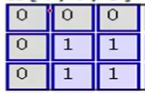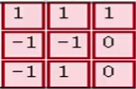对应位置相乘后求和，结果为0

即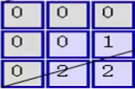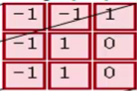对应位置相乘后求和，结果为2

即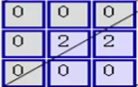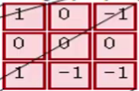对应位置相乘后求和，结果为0

第二步，将3个矩阵产生的结果求和并加上偏置项，即0+2+0+1=3，因此就得到了输出矩阵的左上角的3：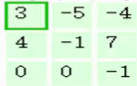第三步，让每一个filter都执行这样的操作，变可得到第一个元素：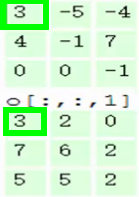第四步，滑动窗口2个步长，重复之前步骤进行计算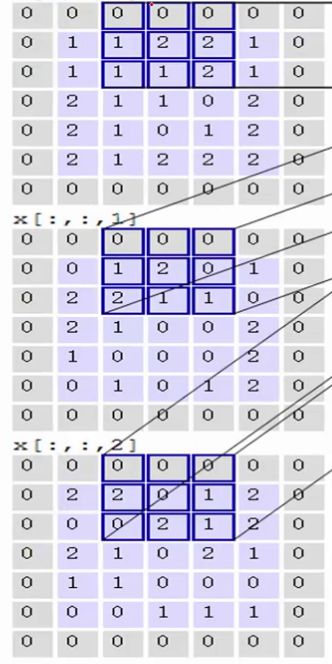第五步，最终可以得到，在2个filter下，卷积后生成的深度为2的输出结果：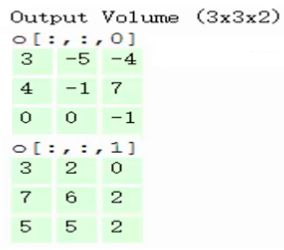1）卷积神经网络卷积后的深度与Filter的个数有关，有几个Filter卷积之后的深度就为几。

2）在计算卷积的过程中，需要进行卷积核翻转。在具体实现上，一般会以互相关操作来代替卷积，从而会减少一些不必要的操作或开销。互相关和卷积的区别仅仅在于卷积核是否进行翻转。因此互相关也可以称为不翻转卷积。在神经网络中使用卷积是为了进行特征抽取，卷积核是否进行翻转和其特征抽取的能力无关。因此，为了实现上（或描述上）的方便起见，我们用互相关来代替卷积。事实上，很多深度学习工具中卷积操作其实都是互相关操作。

3）一次卷积输出的尺寸是多少呢？？？直接参照公式：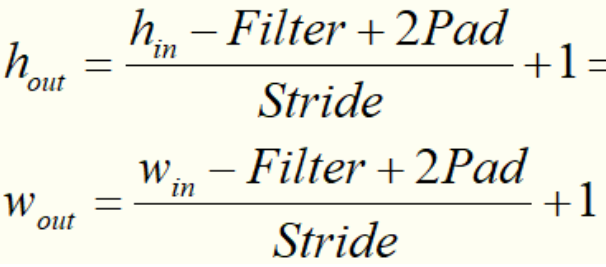2：池化层

用滤波器进行窗口滑动过程中，卷积层实际上"重叠"计算了很多冗余的信息，而池化操作就是去除这些冗余信息，并加快运动（进行特征选择，降低特征数量，并从而减少参数数量）。Pooling的方式其实有多种，一种是取一个区域内所有神经元的最大值。一种是取区域内所有神经元的平均值。

用的最多的是max-pooling就是取一个区域中最大的值，如图将一个4x4的图片max-pooling 一个2x2的图片。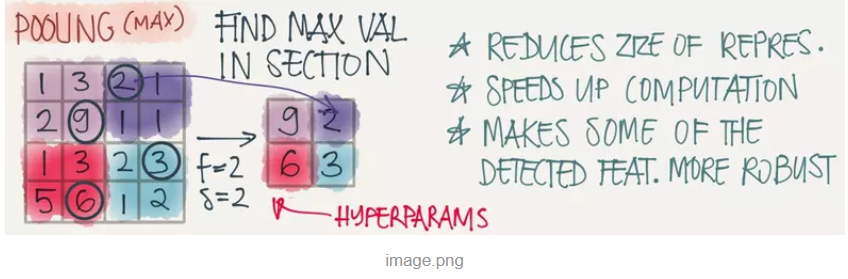### 3.一个完整的深度CNN网络

全连接的DNN，每一层包含一个线性函数和一个激活函数，CNN也一样，在滤波器之后还需要一个激活层，在图像识别应用中，激活层通常用的是Relu函数。线性函数有权重W和偏置b，CNN的权重W就是滤波器的数值，偏置b可以加在Relu之后，一般在池化层之前。

因此一个完整的深度CNN网络，通常由多个卷积层加池化层和最后一个或多个完整层(Full connected(FC))构成，如图：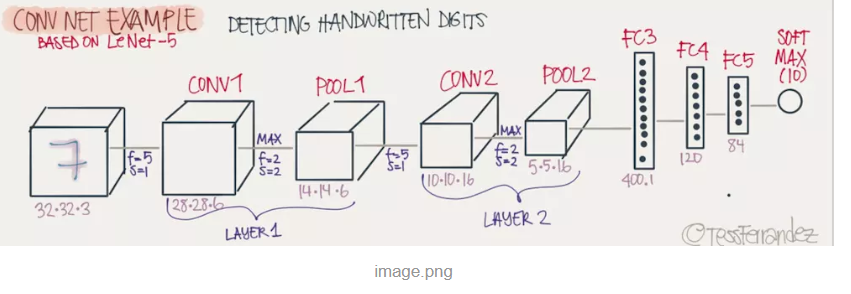1首先：导入需要导入的包,注：函数参数不了解的话可以看pytorch中文文档

``````import torch
import torch.nn as nn
import torch.utils.data as data``````
``import torch.nn.functional as F``
``import matplotlib.pyplot as plt``
``from collections import OrderedDict``

2：然后：简单分为四种方法：

方法1：比较常用。

``````# Method 1 -----------------------------------------
class Net1(torch.nn.Module):
def __init__(self):
super(Net1, self).__init__()
self.conv1 = torch.nn.Conv2d(3, 32, 3, 1, 1)
self.dense1 = torch.nn.Linear(32 * 3 * 3, 128)
self.dense2 = torch.nn.Linear(128, 10)

def forward(self, x):
x = F.max_pool2d(F.relu(self.conv(x)), 2)
x = x.view(x.size(0), -1)
x = F.relu(self.dense1(x))
x = self.dense2(x)
return x

print("Method 1:")
model1 = Net1()
print(model1)'''``````

Method 1:
Net1(
(conv1): Conv2d(3, 32, kernel_size=(3, 3), stride=(1, 1), padding=(1, 1))
(dense1): Linear(in_features=288, out_features=128, bias=True)
(dense2): Linear(in_features=128, out_features=10, bias=True)
)

``'''``

方法二：这种方法利用torch.nn.Sequential（）容器进行快速搭建，模型的各层被顺序添加到容器中。缺点是每层的编号是默认的阿拉伯数字，不易区分。

``````# Method 2 ------------------------------------------
class Net2(torch.nn.Module):
def __init__(self):
super(Net2, self).__init__()
self.conv = torch.nn.Sequential(
torch.nn.Conv2d(3, 32, 3, 1, 1),
torch.nn.ReLU(),
torch.nn.MaxPool2d(2))
self.dense = torch.nn.Sequential(
torch.nn.Linear(32 * 3 * 3, 128),
torch.nn.ReLU(),
torch.nn.Linear(128, 10)
)
def forward(self, x):
conv_out = self.conv1(x)
res = conv_out.view(conv_out.size(0), -1)
out = self.dense(res)
return out

print("Method 2:")
model2 = Net2()
print(model2)
'''
Method 2:
Net2(
(conv): Sequential(
(0): Conv2d(3, 32, kernel_size=(3, 3), stride=(1, 1), padding=(1, 1))
(1): ReLU()
(2): MaxPool2d(kernel_size=2, stride=2, padding=0, dilation=1, ceil_mode=False)
)
(dense): Sequential(
(0): Linear(in_features=288, out_features=128, bias=True)
(1): ReLU()
(2): Linear(in_features=128, out_features=10, bias=True)
)
)
'''``````

``````# Method 3 -------------------------------
class Net3(torch.nn.Module):
def __init__(self):
super(Net3, self).__init__()
self.conv = torch.nn.Sequential()
self.conv.add_module("conv1", torch.nn.Conv2d(3, 32, 3, 1, 1))
self.dense = torch.nn.Sequential()
self.dense.add_module("dense1", torch.nn.Linear(32 * 3 * 3, 128))

def forward(self, x):
conv_out = self.conv1(x)
res = conv_out.view(conv_out.size(0), -1)
out = self.dense(res)
return out

print("Method 3:")
model3 = Net3()
print(model3)
'''
Method 3:
Net3(
(conv): Sequential(
(conv1): Conv2d(3, 32, kernel_size=(3, 3), stride=(1, 1), padding=(1, 1))
(relu1): ReLU()
(pool1): MaxPool2d(kernel_size=2, stride=2, padding=0, dilation=1, ceil_mode=False)
)
(dense): Sequential(
(dense1): Linear(in_features=288, out_features=128, bias=True)
(relu2): ReLU()
(dense2): Linear(in_features=128, out_features=10, bias=True)
)
)
'''``````

``````# Method 4 ------------------------------------------
class Net4(torch.nn.Module):
def __init__(self):
super(Net4, self).__init__()
self.conv = torch.nn.Sequential(
OrderedDict(
[
("conv1", torch.nn.Conv2d(3, 32, 3, 1, 1)),
("relu1", torch.nn.ReLU()),
("pool", torch.nn.MaxPool2d(2))
]
))

self.dense = torch.nn.Sequential(
OrderedDict([
("dense1", torch.nn.Linear(32 * 3 * 3, 128)),
("relu2", torch.nn.ReLU()),
("dense2", torch.nn.Linear(128, 10))
])
)

def forward(self, x):
conv_out = self.conv1(x)
res = conv_out.view(conv_out.size(0), -1)
out = self.dense(res)
return out

print("Method 4:")
model4 = Net4()
print(model4)
'''
Method 4:
Net4(
(conv): Sequential(
(conv1): Conv2d(3, 32, kernel_size=(3, 3), stride=(1, 1), padding=(1, 1))
(relu1): ReLU()
(pool): MaxPool2d(kernel_size=2, stride=2, padding=0, dilation=1, ceil_mode=False)
)
(dense): Sequential(
(dense1): Linear(in_features=288, out_features=128, bias=True)
(relu2): ReLU()
(dense2): Linear(in_features=128, out_features=10, bias=True)
)
)
'''``````
``````# Method 4 ------------------------------------------
class Net4(torch.nn.Module):
def __init__(self):
super(Net4, self).__init__()
self.conv = torch.nn.Sequential(
OrderedDict(
[
("conv1", torch.nn.Conv2d(3, 32, 3, 1, 1)),
("relu1", torch.nn.ReLU()),
("pool", torch.nn.MaxPool2d(2))
]
))

self.dense = torch.nn.Sequential(
OrderedDict([
("dense1", torch.nn.Linear(32 * 3 * 3, 128)),
("relu2", torch.nn.ReLU()),
("dense2", torch.nn.Linear(128, 10))
])
)

def forward(self, x):
conv_out = self.conv1(x)
res = conv_out.view(conv_out.size(0), -1)
out = self.dense(res)
return out

print("Method 4:")
model4 = Net4()
print(model4)
'''
Method 4:
Net4(
(conv): Sequential(
(conv1): Conv2d(3, 32, kernel_size=(3, 3), stride=(1, 1), padding=(1, 1))
(relu1): ReLU()
(pool): MaxPool2d(kernel_size=2, stride=2, padding=0, dilation=1, ceil_mode=False)
)
(dense): Sequential(
(dense1): Linear(in_features=288, out_features=128, bias=True)
(relu2): ReLU()
(dense2): Linear(in_features=128, out_features=10, bias=True)
)
)
'''``````

o

### osc_g8254g7s#### 暂无文章

Vue-插槽slot/具名插槽

12分钟前
6
0

30分钟前
24
0

20
0

17
0

16
0